Prev: 6.1.3.2 Inclusion of Monte Up: 6.1 Modeling of Low-Pressure Next: 6.1.5 Surface Propagation

## 6.1.4 Rate Calculation

For the calculation of local etching or deposition rates, the incident particle distributions have to be evaluated numerically according to the geometric configuration of the structure. This is done by integrating the particle distribution functions over the solid angle visible from all surface positions.

First of all, distribution functions means, that in the general case several species of particles with different distributions and integration limits will be present. Reactive ion etching, e.g., is determined by simultaneous impact of ionized and neutral particles. Moreover particles can have directly incident and reflected distributions originating from different directions. Generally speaking, the final equation for the rate will sum up different fractions with different integration domains.

Secondly, all considered surface positions means, that the surface of the structure has to be discretized. For the cellular geometry representation, the discretized surface is given by the list of material cells which are neighbored by vacuum cells. The accuracy of the volume discretization directly determines the resolution of the surface.

In order to detect the correct integration areas, finding the visible solid angle is a further prerequisite for the rate calculation. For this purpose, the upper hemisphere is scanned by rays starting at a considered surface position, which are tested for intersections with the geometry. For particles directly arriving from the sputter target scanning can be restricted to the upper hemisphere above the wafer. The rays are discretized by the voxels of the cellular representation. If a ray hits a material cell, direct particle incidence from this direction is screened.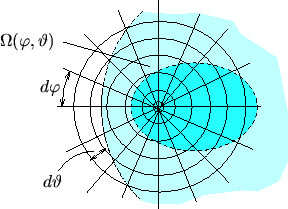For scanning the hemisphere is divided into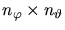bins of size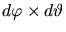(see Fig. 6.7), resulting inscanning operations for each of the approximately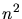surface cells. This number can be reduced by following the limit between visible and shadowed areas, instead of scanning the complete region. When propagating in the polar scanning direction, the azimuthal angle has to be corrected by only a few degrees and the number of operations for each surface cell is reduced fromto approximately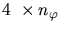, depending on the step width chosen for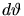. The final result of visibility testing is a discrete function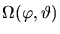which is either 1 for visible directions or 0 for shadowed ones.

Fig. 6.7 shows the polar plot of two common visibility functions. The dark area delimited with the dashed line shows the opening of a circular via seen from an off-center position at the bottom of the via. The light area within the dashed-dotted line shows how a step is seen from a point located at a certain distance away from its lower edge. The polar plot forms a hyperbola reaching its limiting value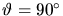for the two polar directions parallel to the step. For the polar angles directed away from the step, where the ray does not intersect with the geometry, the scanning operation is stopped at the bounding box of the simulation domain.

With the visibility functionthe equation for the calculation of the local ratescan be formulated as integral of the distribution function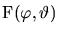and the visibility function. First we only consider the contribution from directly incident particles. Thus the integration can be limited to the upper hemisphere and the formula results in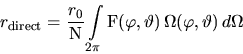(6.6)

where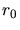is the nominal rate for a flat wafer completely visible to the particle source.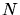is a normalizing factor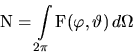(6.7)

which guarantees that for the flat wafer surface the integrated rate equals the nominal rate. Different particle species like ions or neutrals are included with additive terms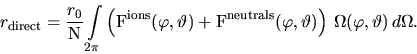(6.8)

Reflections can only occur from the surface of the structure. Therefore the visibility'' function for reflected particles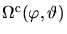is the complement to the visibility function. Reflections also occur from below, e.g., from the bottom of the feature back to the sidewalls. Thus the rate contribution from reflected particles with a separate treatment of ions and neutrals is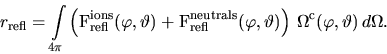(6.9)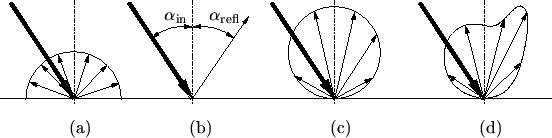Basically, reflection can be classified in four types. The simplest one is diffuse reflection where the distribution of the reflected particles is constant, cf. Fig. 6.8(a). For specular reflection from a certain direction, the surface position, where the particle has been reflected has to be traced back. After applying the reflection law which says that the angle of reflectance is equal to the angle of incidence, cf. Fig. 6.8(b), the reflected intensity from the considered direction is equal to the intensity from the incident direction at the position where the reflection occurs. Additionally, the reflected distribution can be approximated by an analytical expression such as a cosine function, cf. Fig. 6.8(c). Finally, if the reflection process is considered in his overall complexity, the reflected distribution will be composed of specular and diffuse contributions, cf. Fig. 6.8(d). In this case the reflected distribution has to be calculated from the directly incident distribution by applying a transformation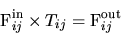(6.10)

which describes the particle-surface interaction of the reflection process and which to determine is a topic of its own.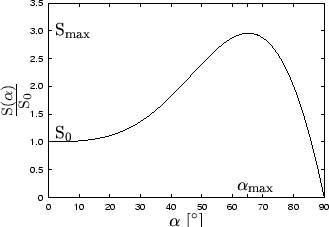Considering reflections means that only a fraction of the directly impinging particles contributes to the rate equation. The sticking coefficient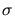denotes the probability that a particle will stick at its initial incidence position without being re-emitted and thus gives the fraction of effective particles.gives the fraction of reflected particles. Hence, the equation of the local ratecombines directly incident (6.8) and reflected (6.9) particles weighted by their fractions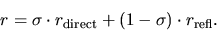(6.11)

Beyond reflections a further interaction term has to be added when the process is determined by angular dependent surface interactions, which is the case when high energetic particles lead to a strongly physically based erosion of the surface . The intensity of the physical surface interaction depends on the direction of the particle incidence with respect to the surface normal and maximum interaction generally occurs for oblique incidence directions. Therefore, yield functions typically show an angular offset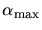for the maximum yield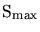with respect to the yield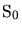for normal incidence, which can be approximated by a linear combination of cosine functions of different orders  as shown in Fig. 6.9.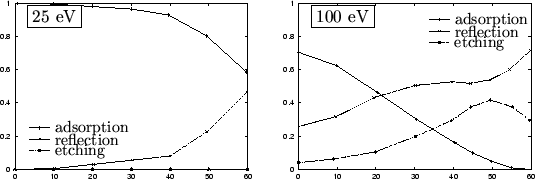Most recently molecular-dynamics simulations of surface interactions in metal film growth  have shown, that adsorption, reflection, and etching processes are occurring simultaneously with different probabilities depending on the angle of incidence. This effect can be taken into consideration by an angular dependent sticking coefficient and by splitting up the distribution functions for the directly incident particles into adsorbed/deposited and etching/reflected fractions which contribute with different sign to the overall surface velocity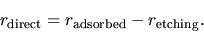(6.12)

The sign of the integrated surface velocityfinally decides, whether the overall effect at the considered surface site is etching or deposition.

It is obvious that the final formula comprises many unknown parameters, like angular dependent particle distributions given separately for adsorption and etching as well as separately for each species, or at least for groups of particles with similar behavior. The list continues with reflection characteristics, the angular dependent sticking coefficients, and the parameters for the sputter yield functions and numerous more. Since many of these parameters are not accessible for measurements and experimental determination of the others is very time consuming, simplified fitting models have to be applied. Before introducing the different models for reactive ion etching, plasma and sputter deposition, the overall model for low-pressure processes will be concluded by the realization of the surface propagation

W. Pyka: Feature Scale Modeling for Etching and Deposition Processes in Semiconductor Manufacturing